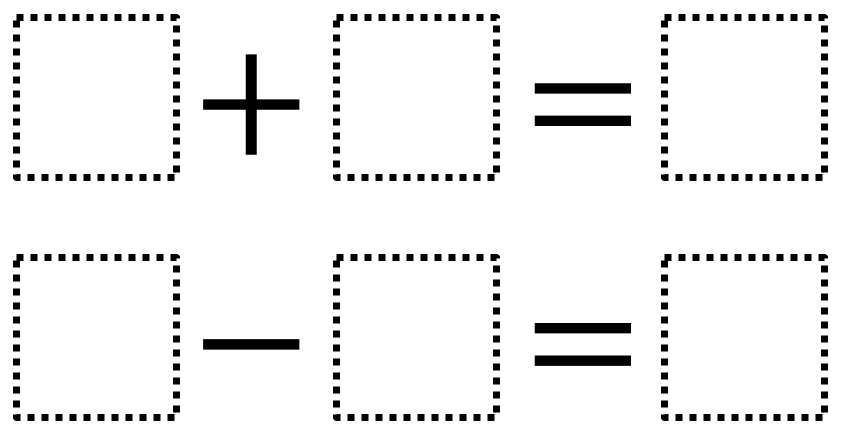# Integer Sums and Differences

Directions: Using the integers -3 to 3, at most one time each, fill in the blanks to make each equation true.### Hint

There are many possibilities. 2 + -3 = -1 and 1 – 3 = -2; 1 + 2 = 3 and -3 – (-1) = -2

Source: Jeanmarie Mullen

## Two Step Inequality with Fractional Coeffcient

Directions: Using the digits 1 to 7 at most one time each, place a digit …

1.The second solution is incorrect. -3 – 1 = -4
It should read -3 – (-1) = -2

•Thanks for pointing this out Kara. I have edited the solution.

•you were correct, if you do a KCC, you get -3 + -1 = -4

2.zero is part of the integer set. Should the directions also state that zero can’t be used?

•I let them use zero. Solutions like -2 + 1 = -1 and 0 – (-3) = 3 were found. Made for some good discussion about the meaning of 0.

3.I really like this activity. Thank you for coming up with it. It is fun and challenging

•It is!! It was fun for my students to figure out!

4.-1+-2= -3

3-2=1
Does this work?

5.3+-3=0
1+-2=-1

6.2+-3=-1
1 -3=-2

7.Richard Buettner

I believe this is an exhaustive list of answers.

-3 + 1 = -2 and 2 – -1 = 3
-3 + 1 = -2 and 2 – 3 = -1
-3 + 2 = -1 and 1 – -2 = 3
-3 + 2 = -1 and 1 – 3 = -2
-3 + 3 = 0 and -1 – -2 = 1
-3 + 3 = 0 and -1 – 1 = -2
-3 + 3 = 0 and 1 – -1 = 2
-3 + 3 = 0 and 1 – 2 = -1
-2 + -1 = -3 and 3 – 1 = 2
-2 + -1 = -3 and 3 – 2 = 1
-2 + 1 = -1 and 0 – -3 = 3
-2 + 1 = -1 and 0 – 3 = -3
-2 + 3 = 1 and -1 – -3 = 2
-2 + 3 = 1 and -1 – 2 = -3
-1 + -2 = -3 and 3 – 1 = 2
-1 + -2 = -3 and 3 – 2 = 1
-1 + 2 = 1 and 0 – -3 = 3
-1 + 2 = 1 and 0 – 3 = -3
-1 + 3 = 2 and -2 – -3 = 1
-1 + 3 = 2 and -2 – 1 = -3
1 + -3 = -2 and 2 – -1 = 3
1 + -3 = -2 and 2 – 3 = -1
1 + -2 = -1 and 0 – -3 = 3
1 + -2 = -1 and 0 – 3 = -3
1 + 2 = 3 and -3 – -2 = -1
1 + 2 = 3 and -3 – -1 = -2
2 + -3 = -1 and 1 – -2 = 3
2 + -3 = -1 and 1 – 3 = -2
2 + -1 = 1 and 0 – -3 = 3
2 + -1 = 1 and 0 – 3 = -3
2 + 1 = 3 and -3 – -2 = -1
2 + 1 = 3 and -3 – -1 = -2
3 + -3 = 0 and -1 – -2 = 1
3 + -3 = 0 and -1 – 1 = -2
3 + -3 = 0 and 1 – -1 = 2
3 + -3 = 0 and 1 – 2 = -1
3 + -2 = 1 and -1 – -3 = 2
3 + -2 = 1 and -1 – 2 = -3
3 + -1 = 2 and -2 – -3 = 1
3 + -1 = 2 and -2 – 1 = -3